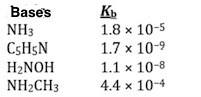# Problem: Using the data in the table, which of the conjugate acids below is the weakest acid? A) NH 3CH 3 + B) H 3NOH + C) C 5H 5NH + D) NH 4 + E) NH 4 + and NH 3CH 3 +

###### Problem Details

Using the data in the table, which of the conjugate acids below is the weakest acid?

A) NH 3CH +

B) H 3NOH +

C) C 55NH +

D) NH +

E) NH + and NH 3CH +What scientific concept do you need to know in order to solve this problem?

Our tutors have indicated that to solve this problem you will need to apply the Conjugate Acids and Bases concept. If you need more Conjugate Acids and Bases practice, you can also practice Conjugate Acids and Bases practice problems.

What is the difficulty of this problem?

Our tutors rated the difficulty ofUsing the data in the table, which of the conjugate acids be...as medium difficulty.

How long does this problem take to solve?

Our expert Chemistry tutor, Dasha took 1 minute and 55 seconds to solve this problem. You can follow their steps in the video explanation above.

What professor is this problem relevant for?

Based on our data, we think this problem is relevant for Professor Goodwin's class at HCC.